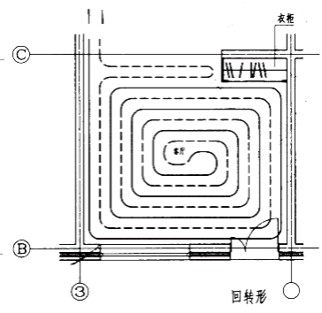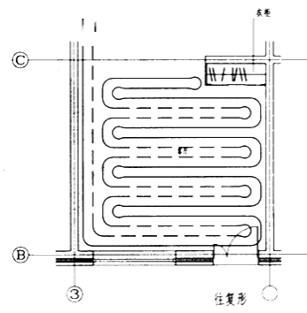HR
【多选题】选择路堤填料时，不得直接使用的土有（）。
A.

B.

C.

D.

E.手机使用分享复制链接新浪微博分享QQ微信扫一扫反馈A.

B.

A.

B.

C.

D.

A.

B.

C.

D.

A.

B.

A.
B.
C.

D.

A.

B.

Q355NDZ25中的Z25表示钢板的沿厚度方向的()为25%。
A.

B.

C.

D.

maximize采用换热器进行分区的高层建筑供暖系统中高低区供水温度相同。
A.

B.

A.

B.

A.

B.

A.

B.

C.

D.

MDA是__。

A.

B.

A.

B.

A.

B.

C.

D.

A.

B.

A.

B.

C.

D.

A.

B.

UML有三种基本构造块，分别是__,__,和 __。

A.

B.

UML模型说明一个系统（）。
A.

B.

C.

D.

304不锈钢相当于我国牌号( )。
A.
108Cr17
B.
30Cr13
C.
06Cr19Ni10
D.
1Cr18Ni9

A.

B.

C.

D.

UML中的事物包括__,__,__,__四种

A.

B.

C.

D.

UML中的关系主要包括__,__,__,__四种。

A.

B.

C.

D.

A.

B.

C.

D.

A.

B.

C.

D.

A.

B.

C.

D.

（）最适合支持描述并行行为，这使之成为支持工作流建模的最好工具。
A.

B.

C.

D.

A.

B.

C.

D.

A.

B.

C.

D.

300系奥氏体不锈钢是( )不锈钢。
A.
Cr-Ni系
B.
Cr-Ni-Mn系
C.
Cr-Mn系
D.
Cr-Mn-Ni-N系
200系奥氏体不锈钢是()不锈钢。
A.
Cr-Ni系
B.
Cr-Ni-Mn系
C.
Cr-Mn系
D.
Cr-Mn-Ni-N系

A.

B.

C.

D.

A.

B.

C.

D.

A.

B.

A.
2.11%
B.
4.9%
C.
2.13%
D.
4.3%

A.

B.

C.

D.

A.B.C.A.

B.

A.

B.

A.

B.

C.

D.

A.

B.

C.

D.

A.

B.

C.

D.

A.

B.

A.

B.

C.

A.
LOC
B.
KLOC
C.
GLOC
D.
ZLOC

A.

B.

A.

B.

C.

D.

A.

B.

C.

D.

A.

B.

C.

D.

A.

B.

A.

B.

A.

B.

C.

D.

A.

B.

A.

B.

A.

B.

A.

B.

C.

D.

A.

B.

C.

D.

A.

B.

C.

D.
V型模型

A.

B.

C.

D.

A.

B.

A.

B.

C.

D.

A.

B.

A.
subversion和git
B.
C.
bugzilla
D.

A.

B.

A.

B.

C.

D.

Photoshop处理图像之前，首先必须打开该图像，以下哪些方式能够使Photoshop正确打开图像？
A.

B.

C.

D.

Photoshop的文档窗口包含图片的哪些文件信息？
A.

B.

C.

D.

E.

A.

B.

A.

B.

C.

D.

A.

B.

C.

D.

A.

B.

A.

B.

C.

D.

A.

B.

C.

D.

A.

B.

C.

A.

B.

Photoshop的菜单栏包含各种可以执行的命令，通过单击左键菜单即可打开菜单及选择需要的命令。每个菜单包含多个命令，其中有些命令带有 “箭头”符号，表示该命令还有多个子命令；有些命令后面带有一连串的字母或字母组合，表示是Photoshop命令相应的（ ）
A.

B.

C.

D.

A.
Q1=Q2=Q3
B.
Q1>Q2>Q3
C.
Q1<Q2<Q3
D.
Q1=Q2<Q3
HT100是以()为基体和粗片状的石墨组成的普通灰铸铁。
A.

B.

C.

D.

A.

B.

C.

D.

HT200、HT225、HT250是以()为基体和中等片状的石墨组成的灰铸铁。
A.

B.

C.

D.

A.

B.

C.

D.

HT150是以()为基体和粗片状的石墨组成的普通灰铸铁。
A.

B.

C.

D.

A.

B.

C.

D.

A.

B.

C.

D.

A.

B.294%